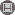Volume 14, Issue 2 (10-2019)                   IJMSI 2019, 14(2): 79-92 | Back to browse issues page

BibTeX | RIS | EndNote | Medlars | ProCite | Reference Manager | RefWorks
Send citation to:Gao Z, Han R, Lee S, Ren H, Lau G. Labeling Subgraph Embeddings and Cordiality of Graphs. IJMSI. 2019; 14 (2) :79-92
URL: http://ijmsi.ir/article-1-925-en.html
Abstract:

Let \$G\$ be a graph with vertex set \$V(G)\$ and edge set \$E(G)\$, a vertex labeling \$f : V(G)rightarrow mathbb{Z}_2\$ induces an edge labeling \$ f^{+} : E(G)rightarrow mathbb{Z}_2\$ defined by \$f^{+}(xy) = f(x) + f(y)\$, for each edge \$ xyin E(G)\$.  For each \$i in mathbb{Z}_2\$, let \$ v_{f}(i)=|{u in V(G) : f(u) = i}|\$ and \$e_{f^+}(i)=|{xyin E(G) : f^{+}(xy) = i}|\$. A vertex labeling \$f\$ of a graph \$G\$ is said to be friendly if \$| v_{f}(1)-v_{f}(0) | leq 1\$. The friendly index set of the graph \$G\$, denoted by \$FI(G)\$, is defined as  \${|e_{f^+}(1) - e_{f^+}(0)|\$ : the vertex labeling \$f\$ is friendly\$}\$. The full friendly index set of the graph \$G\$, denoted by \$FFI(G)\$, is defined as \${e_{f^+}(1) - e_{f^+}(0)\$ : the vertex labeling \$f\$ is friendly\$}\$. A graph \$G\$ is cordial if \$-1, 0\$ or \$1in FFI(G)\$. In this paper, by introducing labeling subgraph embeddings method, we determine the cordiality of a family of cubic graphs which are double-edge blow-up of \$P_2times P_n, nge 2\$. Consequently, we completely determined friendly index and full product cordial index sets of this family of graphs.

Type of Study: Research paper | Subject: Special